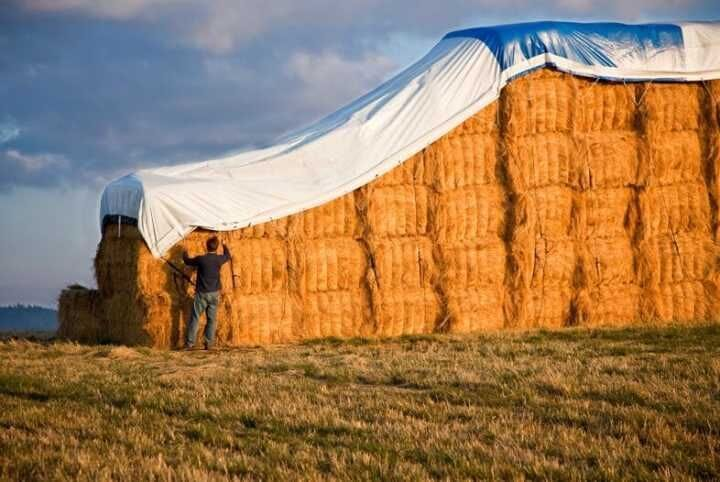### What is the density of tarpaulin?

26 Jun 2019

Author:

Source:

The calculation unit of tarpaulin fabric density is in metric, which refers to the number of warp and weft yarns arranged within 10 cm. The density directly affects the appearance, feel, thickness, strength, folding resistance, breathability, wear resistance and thermal insulation properties of the fabric, and it also relates to the cost and productivity of the product.

(One)

Indirect test

This method is suitable for fabrics of a regular structure with a high density and a small number of yarns. First, after analyzing the number of warp yarns in the fabric and its tissues

(The number of tissue loops), then multiplied by the number of tissue loops in 10 cm, and the resulting product is the warp (weft) yarn density.(two)

Direct measurement

Direct measurement is done by means of a mirror or fabric density analysis mirror. The tarp fabric density analysis mirror has a scale length of 5cm. Under the analysis lens, a long strip of glass is engraved with a red line. When analyzing the density of the fabric, move the lens to align the red line on the glass sheet with the red line on the scale. Between the root yarns, use this as a starting point, and move the number of yarns on the side of the lens until the 5cm mark is used. The number of yarns output is multiplied by 2, which is the density value of the 10 cm fabric.

When counting the number of yarns, the center between the two yarns should be used as the starting point. If the number reaches the end point, it exceeds 0.5, and if less than one, it should be calculated according to 0.75.

If it is less than 0.5, it is calculated as 0.25. The fabric density should generally be measured from 3-4 data, and then the arithmetic mean is taken as the measurement result.